In a reaction,

${\mathrm{CH}}_{2}={\mathrm{CH}}_{2}$$\underset{\mathrm{acid}}{\overset{\mathrm{Hypochlorous}}{\to }}$M$\stackrel{\mathrm{R}}{\to }$$\underset{{\mathrm{CH}}_{2}\mathrm{OH}}{\underset{|}{{\mathrm{CH}}_{2}\mathrm{OH}}}$

M = molecules, R = reagent. M and R are

1. ${\mathrm{CH}}_{3}{\mathrm{CH}}_{2}\mathrm{Cl}$ and NaOH

2. ${\mathrm{CH}}_{2}\mathrm{Cl}.{\mathrm{CH}}_{2}\mathrm{OH}$ and aq. ${\mathrm{NaHCO}}_{3}$

3. ${\mathrm{CH}}_{3}{\mathrm{CH}}_{2}\mathrm{OH}$ and HCl

4.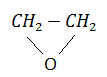and heat

Concept Questions :-

Alkanes, Alkenes and Alkynes -Methods of Preparation
High Yielding Test Series + Question Bank - NEET 2020

Difficulty Level:

When 3,3-dimethyl-2-butanol is heated with conc.${\mathrm{H}}_{2}{\mathrm{SO}}_{4}$, the major product obtained is

1. 2,3-dimethyl-2-butene

2. 3,3-dimethyl-2-butene

3. 2,3-dimethyl-1-butene

4. cis and trans-isomers of 2,3-dimethyl-2-butene

Concept Questions :-

Alkanes, Alkenes and Alkynes - Chemical Properties
High Yielding Test Series + Question Bank - NEET 2020

Difficulty Level:

During ozonolysis of ${\mathrm{CH}}_{2}={\mathrm{CH}}_{2}$ if hydrolysis is made in absence of Zn dust the products formed are:

1. HCHO                                  2. HCOOH

3. ${\mathrm{CH}}_{3}\mathrm{OH}$                                4. ${\mathrm{CH}}_{2}{\mathrm{OHCH}}_{2}\mathrm{OH}$

Concept Questions :-

Alkanes, Alkenes and Alkynes - Chemical Properties
High Yielding Test Series + Question Bank - NEET 2020

Difficulty Level:

${\mathrm{CH}}_{3}\mathrm{C}\equiv \mathrm{C}.{\mathrm{CH}}_{3}$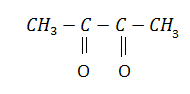In the above reaction X is:

(a) ${\mathrm{HNO}}_{3}$                                (b) ${\mathrm{O}}_{2}$

(c) ${\mathrm{O}}_{3}$                                     (d) ${\mathrm{KMnO}}_{4}$

Concept Questions :-

Alkanes, Alkenes and Alkynes - Chemical Properties
High Yielding Test Series + Question Bank - NEET 2020

Difficulty Level:

Major product of the following reaction is: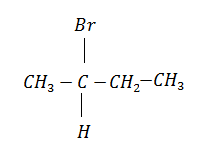+ Alc.KOH$\to$ ?

(a) butene-1                                  (b) butene-2

(c) butane                                     (d) butyne-1

Concept Questions :-

Alkanes, Alkenes and Alkynes - Chemical Properties
High Yielding Test Series + Question Bank - NEET 2020

Difficulty Level:

An alkene, obtained by the dehydration of an alcohol (A), on ozonolysis gives two molecules of acetaldehyde for every molecule of alkene. The alcohol (A) is:

(a) ${\mathrm{CH}}_{3}{\mathrm{CH}}_{2}{\mathrm{CH}}_{2}\mathrm{OH}$                             (b) ${\mathrm{CH}}_{3}{\mathrm{CH}}_{2}\mathrm{OH}$

(c) ${\mathrm{CH}}_{3}\mathrm{CH}={\mathrm{CHCH}}_{2}\mathrm{OH}$                       (d)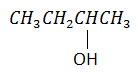Concept Questions :-

Alkanes, Alkenes and Alkynes - Chemical Properties
High Yielding Test Series + Question Bank - NEET 2020

Difficulty Level:

Gammexane (a $\mathrm{\gamma }$-isomer) is:

1. BHC                                          2. benzene hexachloride

3. lindane                                      4. all of these

High Yielding Test Series + Question Bank - NEET 2020

Difficulty Level:

In the following reaction the catalyst used is: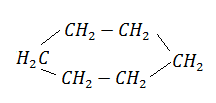$\to$${\mathrm{C}}_{6}{\mathrm{H}}_{6}$ + 3${\mathrm{H}}_{2}$

1. ${\mathrm{Cr}}_{2}{\mathrm{O}}_{3}$                                      2. $A{l}_{2}{\mathrm{O}}_{3}$

3. Zn dust                                    4. ${\mathrm{Cr}}_{2}{\mathrm{O}}_{3}$ and $A{l}_{2}{\mathrm{O}}_{3}$

Concept Questions :-

Alkanes, Alkenes and Alkynes - Chemical Properties
High Yielding Test Series + Question Bank - NEET 2020

Difficulty Level:

Silver acetylide when heated with HCl gives:

(a) ${\mathrm{C}}_{2}{\mathrm{H}}_{2}$                                (b) ${\mathrm{H}}_{2}$

(c) ${\mathrm{C}}_{2}{\mathrm{H}}_{4}$                                (d) ${\mathrm{C}}_{6}{\mathrm{H}}_{6}$

High Yielding Test Series + Question Bank - NEET 2020

Difficulty Level:

$\mathrm{R}-{\mathrm{CH}}_{2}-{\mathrm{CCl}}_{2}-\mathrm{R}\stackrel{\mathrm{Reagent}}{\to }$$\mathrm{R}-\mathrm{C}\equiv \mathrm{C}-\mathrm{R}$. The reagent is

1. Na                                     2. HCl in ${\mathrm{H}}_{2}\mathrm{O}$

3. KOH in ${\mathrm{C}}_{2}{\mathrm{H}}_{5}\mathrm{OH}$                 4. Zn in alcohol

Concept Questions :-

Alkanes, Alkenes and Alkynes - Chemical Properties